# Invasive Weed Optimization Algorithm for Tuning Transitioning from Type-1 to Interval Type-2 Fuzzy Logic Controller for Boost DC-DC Converters

Invasive Weed Optimization Algorithm for Tuning Transitioning from Type-1 to Interval Type-2 Fuzzy Logic Controller for Boost DC-DC Converters
Laboratoire des semi-coducteurs et des matériaux fonctionnels, University of Amar Telidji, Laghouat 03000, Algeria

University of Ziane Achour, Djelfa 17000, Algeria

Corresponding Author Email:
a.bennaoui@lagh-univ.dz
Page:
195-202
|
DOI:
https://doi.org/10.18280/jesa.530205
19 January 2020
|
Accepted:
5 March 2020
|
Published:
20 May 2020
| Citation

OPEN ACCESS

Abstract:

Some studies tried to make transition from Type-1 to interval Type-2 membership functions, but they get the problems of choosing the footprint uncertainty size in the Interval Type-2 Membership Functions. In this paper, our objective is to employ two optimization methods: Invasive Weed Optimization (IWO) and Particle Swarm Optimization (PSO) for tuning the Transitioning from Type-1 To Interval Type-2 Fuzzy Logic Controller for Boost DC-DC Converters and compare their performances. Also, we will discuss the effects of the PID values in the operation of transition from Type-1 to interval Type-2 fuzzy logic Controller for Boost DC-DC Converters. The simulation results show IWO optimization methods is helpful to Tuning the Transitioning from Type-1 To Interval Type-2 Fuzzy Logic Controller for Boost DC-DC Converters. Moreover, when we tune both PID values and the FOU size in T2-MFs of Interval Type-2 Fuzzy Logic PID-controller, we will get the best performance for interval Type-2 Fuzzy Logic PID-controller for Boost DC-DC Converter. To sum up; the optimal footprint of uncertainty (FOU) size in interval Type-2 membership functions, they have an essential role and good effect in the performance of Interval Type-2 fuzzy logic Controller for Boost DC-DC Converters.

Keywords:

IWO, Type-2 fuzzy logic controller, Type-1 membership functions, interval Type-2 membership functions, footprint of uncertainty (FOU)

1. Introduction

The membership functions (MFs) enable establishing a relationship between numerical values and linguistic labels.

Type-1 fuzzy MFs (T1-MF) are two dimensional and represent the membership degree μ for a variable x. Type-2 fuzzy MFs (T2-MF) are three dimensional:

They consider an uncertainty U of the membership degree. T1-MFs are a particular case of T2-MFs where the uncertainty value is 0. Membership functions are classified as (Figure 1 and Figure 2):

The concept of Type-2 fuzzy set was initially proposed as an extension ofType-1 fuzzy set by Prof. Zadeh .

## 1.1.pngFigure 1. Membership functions (a) singleton, (b) Interval Type-1, (c) Type-1

## 1.2a.png(a)

## 1.2b.png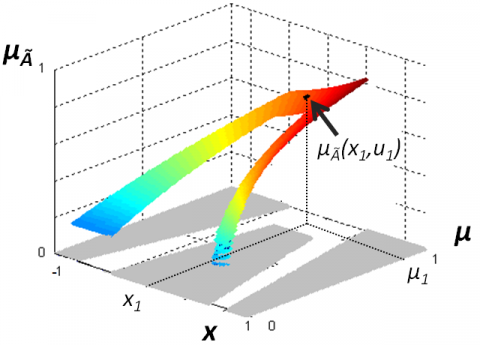(b)

Figure 2. Membership functions (a) interval Type-2, (b) general Type-2

The Type-2 fuzzy system is characterized by a fuzzy membership function, i.e., the membership grade for each element of this set is a fuzzy set in [0,1], contrary a Type-1 fuzzy set where the membership grade is a crisp number in [0,1]. Such sets are very useful in circumstances where they are difficult to determine an exact membership function for a fuzzy set; hence, they are useful for incorporating uncertainties. Type-2 fuzzy sets are appropriate for modeling uncertainty as Type-2 fuzzy sets include FOU (Footprint of Uncertainty) and third dimension, offering extra degrees of freedom to Type-2 fuzzy sets in comparison to Type-1 fuzzy sets [2, 3].

The performance of Type-2 Fuzzy Logic Controller (T1 FLC) is affected by the Footprint of uncertainty size in interval Type-2 membership functions [4-8].

Some studies propose [7, 8] to transition from type-l to interval Type-2 fuzzy sets, through varying the size FOU (Footprint of uncertainty) in interval Type-2 membership functions, but there are problems of tuning the footprint of uncertainty size parameter in The Interval Type-2 Membership Functions.

In this work, we developed a new approach to transit from Type-1 to interval Type-2 fuzzy logic Controller using the optimization methods (IWO and PSO). Also, we used the optimization methods (IWO and PSO) for tuning the PID values and the footprint uncertainty size in the Interval Type-2 Membership Functions of Interval Type-2 Fuzzy Logic PID Controller for Boost DC-DC Converters.

This paper will be organized as following:

Section 2: Converter modeling. section 3: The Type-2 TSK Fuzzy Logic Controller. section 4: Invasive Weed Optimization Algorithm. section 5: Fuzzy PID Controller for Boost DC-DC Converters. section 6: Simulation Phases and Results. section 7: Conclusion.

2. The DC–DC Boost Converter

In this study we will use the DC–DC Boost converter (Figure 3 and Table 1).

## 1.3.pngFigure 3. The DC–DC boost converter

Table 1. The parameters of the DC–DC boost converter

 the parameters of the DC–DC Boost converter Series Inductance $\mathrm{L}=20[\mathrm{mH}]$. Parallel Capacitance $\mathrm{C}=20[\mu \mathrm{f}]$ load resistance $\mathrm{R}=30[\Omega]$ Input Voltage $\mathrm{Vg}=15[\mathrm{V}]$ Switching frequency $\mathrm{f}_{\mathrm{SW}}=5[\mathrm{kHz}]$.

Vg: Represents power supply voltage. iL: The current through the inductance L. sw: An electronic switch. VD: Voltage of the diode. Vc: The voltage on the capacitor C. u0(t) Voltage output across the resistive load R.

Continuous conduction mode (CCM) has two topologies depending on the position of switch swand. In this simulation, we use the Boost DC-DC Converter operating in continuous conduction mode (CCM).

The following equations represent the first topology (Figure 4) of DC–DC Boost converter:

$L \frac{d}{d t} \mathrm{i}_{L}(t)=V_{\mathrm{g}}(t)$

$\frac{d}{d t} v_{\mathrm{c}}(t)=-\frac{1}{C R} v_{c}(t)$      (1)

$u_{0}(t)=v_{c}(t)$

## 1.4.pngFigure 4. The first topology (sw closed, VD opened)

The following equations represent the second topology (Figure 5) of DC–DC Boost converter:

## 1.5.pngFigure 5. The second topology (sw opened, VD closed)

$L \frac{d}{d t} \mathrm{i}_{L}(t)=v_{\mathrm{g}}(t)-v_{c}(t)$

$\frac{d}{d t} v_{c}(t)=\frac{1}{C R}\left(R \mathrm{i}_{L}(t)-v_{\mathrm{c}}(t)\right)$       (2)

$u_{0}(t)=v_{c}(t)$

The state equation of the boost DC-DC converter can be stated as :

$\dot{x}=A_{i} x+B_{i} V_{\mathrm{g}}(t)$

$u_{0}=C_{i} x$ with $x=\left[i_{L} v_{\mathrm{c}}\right]^{T}$       (3)

where, subscript 1 stands for transistor ON, and subscript 2 stands for transistor OFF of the converter circuit.

$A_{i}, B_{i}$ and $C_{i}$ are system Matrices of the constituent linear circuits.

The system matrices can be obtained for different operating modes as:

$C_{1}=[0 \quad 1], C_{2}=[0 \quad 1] \cdot A_{1}=\left[\begin{array}{cc}0 & 0 \\ 0 & -\frac{1}{R C}\end{array}\right],$ and $A_{2}=$$\left[\begin{array}{cc}0 & -\frac{1}{L} \\ \frac{1}{c} & -\frac{1}{R C}\end{array}\right] \cdot B_{1}=B_{2}=\left[\frac{1}{L} 0\right]^{T} The State-Space Averaged model represented in the following equations [10-13]: \left\{\begin{array}{c}\dot{x}=A_{a v g} x+B_{a v g} u \\ a n d \\ y=C_{a v g} x\end{array}\right. (4) where, \left\{\begin{array}{l}A_{a v g}=d A_{1}+(1-d) A_{2} \\ B_{a v g}=d B_{1}+(1-d) B_{2} \\ C_{a v g}=d C_{1}+(1-d) C_{2}\end{array}\right. 3. The Type-2 TSK Fuzzy Logic Controller A Type-2 TSK fuzzy logic controller was firstly introduced by Mendel and Liang. We have three models of T2 TSK fuzzy logics based on the type of the antecedent and consequent part of rules [14-16] (Table 2 and Table 3). Table 2. Classification of other t2 tsk fls models [15, 16]  Antecedent Consequent The Rule Base T2 TSK FLS Model I Type-2 fuzzy sets Type-1 fuzzy sets IF \mathrm{x}_{1} is \tilde{\mathrm{F}}_{1}^{\mathrm{i}} and... \mathrm{x}_{\mathrm{p}} is \tilde{\mathrm{F}}_{\mathrm{p}}^{\mathrm{i}} THEN \mathrm{y}^{1}=\mathrm{C}_{0}^{1}+\mathrm{C}_{1}^{1} \mathrm{x}_{1} \ldots+\mathrm{C}_{\mathrm{p}}^{1} \mathrm{x}_{\mathrm{p}} T2 TSK FLS Model II Type-2 fuzzy sets Crisp Numbers IF \mathrm{x}_{1} is \tilde{\mathrm{F}}_{1}^{\mathrm{i}} and... \mathrm{x}_{\mathrm{p}} is \tilde{\mathrm{F}}_{\mathrm{p}}^{\mathrm{i}} THEN \mathrm{y}^{1}=\mathrm{C}_{0}^{1}+\mathrm{C}_{1}^{1} \mathrm{x}_{1} \ldots+\mathrm{C}_{\mathrm{p}}^{1} \mathrm{x}_{\mathrm{p}} T2 TSK FLS Model III Type-2 fuzzy sets Type-1 fuzzy sets IF \mathrm{x}_{1} is \tilde{\mathrm{F}}_{1}^{\mathrm{i}} and... \mathrm{x}_{\mathrm{p}} is \tilde{\mathrm{F}}_{\mathrm{p}}^{\mathrm{i}} THEN \mathrm{y}^{1}=\mathrm{C}_{0}^{1}+\mathrm{C}_{1}^{1} \mathrm{x}_{1} \ldots+\mathrm{C}_{\mathrm{p}}^{1} \mathrm{x}_{\mathrm{p}} Table 3. The final output of other T2 TSK FLS models  The final output Of T2 TSK Models T2 TSK FLS- Model I The final output is also an interval Type-1 set and is calculated as follows [15-17]: Y\left(Y^{1}, \ldots Y^{M}, F^{1}, \ldots, F^{M}\right)=\left[y_{l}, y_{r}\right]=\int_{y^{1}} \ldots \int_{y^{M}} \int_{f^{1}} \ldots \int_{f^{M}} 1 / \frac{\sum_{i=1}^{M} f^{i} y^{i}}{\sum_{i=1}^{M} f^{i}} Where M is the number of rules fired, y_{i} \in Y^{i}, and Y^{i}=\left[ y_{1}^{i}, y_{r}^{i}\right],(i=1 \ldots M) T2 TSK FLS -Model II The final output Is a special case of (5), because now each Y^{i} is a crisp value y^{i}. So Y\left(f^{1}, \ldots, f^{M}\right)=\left[y_{l}, y_{r}\right]=\int_{f^{1}} \ldots \int_{f^{M}} 1 / \frac{\sum_{i=1}^{M} f^{i} y^{i}}{\sum_{i=1}^{M} f^{i}} T2 TSK FLS -Model III The final output is special case of (5), because now each F^{i} is a crisp value f^{i} So Y\left(Y^{1}, \ldots Y^{M}\right)=\left[y_{l}, y_{r}\right]=\int_{y^{1}} \ldots \int_{y^{M}} 1 / \frac{\sum_{i=1}^{M} f^{i} y^{i}}{\sum_{i=1}^{M} f^{i}} where, i=1,2, \ldots \ldots \ldots, M, C_{k}^{i}(k=1,2, \ldots, p) are the consequent parameters whitch are Type-1 fuzzy set, c_{k}^{i}(k=1,2, \ldots, p) are the consequent parameters that are crisp numbers, Y^{l} are the outputs of the l^{\text {th }} rule, \tilde{F}_{j}^{i}(j=1 \ldots . . p \text { ) are Type- } 2 fuzzy sets of input state j in rule M, given an inputs x_{1}, x_{2} \ldots \ldots x_{p}, F_{j}^{i}(j=1 \ldots \ldots p) are Type- 1 fuzzy sets. The firing strength of the ith rule F^{i}(x) with meet operation under product or minimum t-norm is an interval Type-1 set expressed as: F^{i}(x)=\left[\underline{f}^{i}(x), \bar{f}^{i}(x)\right] (5) where, \underline{f}^{i}(x)=\underline{\mu}_{\tilde{F}_{1}^{i}}\left(x_{1}\right) * \ldots \underline{\mu}_{\tilde{F}_{p}^{i}}\left(x_{p}\right) \bar{f}^{i}(x)=\bar{\mu}_{\tilde{F}_{1}^{i}}\left(x_{1}\right) * \ldots \bar{\mu}_{\tilde{F}_{p}^{i}}\left(x_{p}\right) To compute Y it is only necessary to compute its two end-points y1 and yr can also be computed more efficient by the KM Algorithm and the Defuzzified output is: y=\frac{y_{l}+y_{r}}{2} (6) 4. Invasive Weed Optimization Algorithm Invasive weed optimization (IWO) was developed by Mehrabian and Lucas in 2006 [18, 19], The invasive weed optimization technique is a population-based evolutionary optimization method inspired by the behavior of weed colonies, Invasive weed optimization technique has been successfully used to a variety of optimization problems [20-25]. The process is addressed in these steps (Figure 6): ## 1.6.png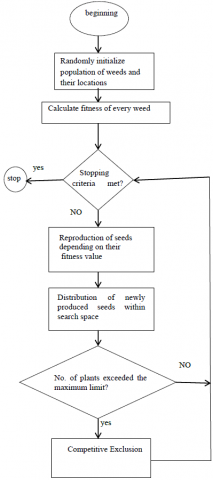Figure 6. Invasive weed optimization algorithm flowchart The procedure starts off evolved with initializing a population. Its capacity that a populace of preliminary options is randomly generated over the problem space. Then contributors of the population produce seeds relying on their relative fitness in the population. In other words, the range of seeds for every member is starting with the value of \mathrm{S}_{\min } for the worst member and increases linearly to \mathrm{S}_{\max } for the first-rate member. For next step, these seeds are randomly scattered over the search area by way of generally distributed random numbers with mean equal to zero and an adaptive standard deviation [18, 19]. the standard deviation (SD) [18, 19] for every generation is in: \sigma_{\text {iter }}=\frac{\left(\text { iter }_{\text {max }}-\text { iter }\right)^{\mathrm{n}}}{\left(\text { iter }_{\text {max }}\right)^{\mathrm{n}}}\left(\sigma_{\text {init }}-\sigma_{\text {final }}\right)+\sigma_{\text {final }} (7) n: is the nonlinear modulation index, \sigma_{\text {iter }} is the standard deviation at the current iteration and iter _{\max }: is the maximum number of iterations. The produced seeds, accompanied through their parents are viewed as the practicable solutions for the subsequent generation. Finally step, a competitive exclusion is conducted in the algorithm, i.e., after a number of iterations the population reaches its maximum, and an elimination mechanism have to be employed. To this end, the seeds and their parents are ranked together and those with better fitness live on and end up reproductive [18, 19]. 5. Fuzzy PID Controller for Boost DC-DC Converters Fuzzy PID Controller systems (Figure 7 and Figure 8) have double inputs and single output. The error (e) and the change of error (de) are used as the inputs and the change of the control signal \widehat{d_{1}} is used as the output of the FLC ## 1.7.png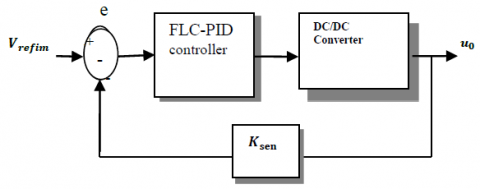Figure 7. The FLC-PID controller for boost dc-dc converters ## 1.8.pngFigure 8. Structure of a fuzzy logic PID Controller (FLC-PID) K_{\mathrm{pi}}, K_{\mathrm{pd}} are values of PID. G_{1}, G_{2} values of the gains normalization of the fuzzy system inputs. \text { where, } G_{1}=0.5 \text { and } G_{2}=9 The reference voltage: \operatorname{Vref}=37.5 \mathrm{V}. sensor of gain: K_{\operatorname{sen}}=0.04 And V_{\text {refim }}=K_{\text {sen }} * V_{\text {ref }}, e=V_{\text {refim }}-K_{\text {sen }} * u_{0} This system is constructed from the human experience formulated in a collection of fuzzy rules in the following form: \mathrm{j}^{\mathrm{th}}: \mathrm{IF} \mathrm{e} is \mathrm{E}_{0}^{\mathrm{j}} and \operatorname{de} is \mathrm{E}_{1}^{\mathrm{j}} \mathrm{THEN} \hat{\mathrm{d}}_{1}=\mathrm{C}_{\mathrm{j}}(\mathrm{e}, \mathrm{de}) With \mathrm{E}_{0}^{\mathrm{j}} \mathrm{E}_{1}^{\mathrm{j}} are respectively the fuzzy sets of the error voltage e and its time derivative de C_{\mathrm{j}} is the \mathrm{j}^{\text {th }} output singleton. The strategy of fuzzy control is derived using the following knowledge on the system: The change of duty cycle \hat{\mathrm{d}}{1} must be large, when u_{0} is far from the reference. Vreffor provides a small response time. The small change of duty cycle \hat{\mathrm{d}}{1} is sufficient to reach the reference providing that u_{0} approaches the reference. The duty cycle must be unchanged as long as u_{0} is in the vicinity of the reference with a sufficient approaching speed, for preventing the output overshoot. When u_{0} reaches the reference and continue growing up: first, we decrease the duty cycle change, then if u_{0} remains closer to the reference, the duty cycle changes must be zero otherwise, it must be negative. Thus, the final control action \hat{\mathrm{d}} applied to the converter is given by: \hat{\mathrm{d}}=\mathrm{G}_{1} \hat{\mathrm{d}}_{1}+\mathrm{G}_{2} \int \widehat{\mathrm{d}}_{1} \mathrm{d} (8) We obtain 25 fuzzy rules with 17 output singletons issued from the human expertise (Table 4). Table 4. Rules and output membership functions [8, 26]  e\de PH PL Z NL NH PH 1 0.81 0.49 0.36 0.25 PL 0.64 0.36 0.16 0.04 0 Z 0.16 0.04 0 -0.04 -0.16 NL 0 -0.04 -0.16 -0.36 -0.64 NH -0.25 -0.36 -0.49 -0.81 -1 6. Simulation Phases and Results In this work, we propose using the optimizations methods (IWO and PSO) to tuning the footprint of uncertainty size (FOU) in interval Type-2 fuzzy sets. Besides; We discuss the effects of the PID values in the operation of transition from Type-1 to interval Type-2 fuzzy logic Controller for Boost DC-DC Converters (Figure 9). ## 1.9.pngFigure 9. The FLC-PID controller with optimizations methods We use the two inputs fuzzy sets (e and de) are composed of five membership functions [8, 26] are defined in (Figure 10). ## 1.10a.png## 1.10b.pngFigure 10. The two inputs fuzzy sets (e and de) The transition from Type-1 membership functions to interval Type-2 membership functions shown in (Figure 11). [7, 8, 27]. The uncertainty size parameter (U) in the interval Type-2 membership functions is to be determined and optimized, using optimization algorithms (IWO and PSO). ## 1.11.pngFigure 11. Interval Type-2 membership functions [7, 8, 26] After make transition from Type-1membership functions (Figure 12) to interval Type-2 membership functions ## 1.12.pngFigure 12. Type-1 membership functions where, \Delta \mathbf{x}_{1}=\mathbf{x}_{2}-\mathbf{x}_{1} ; \Delta \mathbf{x}_{2}=\mathbf{x}_{3}-\mathbf{x}_{2} The uncertainty (U) in the membership functions is the third considered parameter. we need to avoid overlapping between: \mathrm{x}_{1}^{\text {lower }} and \mathrm{x}_{3}^{\text {lower }}, so, we propose: \mathrm{U} \in[\mathbf{0}, \mathbf{1}] \Rightarrow \Delta \mathbf{x}_{1} * \mathbf{U} \leq$$\Delta \mathbf{x}_{1}$ and $\Delta \mathbf{x}_{2} * \mathbf{U} \leq \Delta \mathbf{x}_{2}$

$x_{1}^{\text {Upper }}=\max \left(-1, x_{1}-\Delta x_{1} * \mathbf{U} / 2\right)$.

$x_{1}^{l o w e r}=\max \left(-1+\Delta x_{1} * \mathbf{U}, x_{1}+\Delta x_{1} * \mathbf{U} / 2\right)$.

$x_{3}^{\text {lower }}=\min \left(1-\Delta x_{2} * \mathbf{U}, x_{3}-\Delta x_{2} * \mathbf{U} / 2\right)$.

$x_{3}^{\text {Upper }}=\min \left(x_{3}+\Delta x_{2} * \mathbf{U} / 2,1\right)$.

The uncertainty size Parameter (U) is to be determined and optimized, using optimization algorithms (IWO and PSO).

The objective function as following:

$\operatorname{cost}$ function $=\frac{\left(10^{3}\right)}{n} \sum_{t=0}^{n}[e(t)]^{2}$      (9)

In this work we have two types of simulation.

Firstly: tuning the FOU size in T2-MFsof Interval Type-2 Fuzzy Logic PID controller.

where,

1. we make transitioning from Type-1 to Interval Type-2 Fuzzy Logic controller and optimizing the FOU size ($\mathrm{U} \in[0,1]$) in interval Type-2 membership functions using optimization algorithms (IWO and PSO).
2. The PID gains $\left(K_{p d} \text { And } K_{p i}\right)$ are given  $K_{p d}=0.25, K_{p i}=255$

Secondly: tuning both the PID values and the FOU size in T2-MFsof Interval Type-2 Fuzzy Logic PID controller.

where,

1. We make transition from Type-1 to Interval Type-2 Fuzzy Logic controller and Optimizing the FOU size ($\mathrm{U} \in[0,1]$) in interval Type-2 membership functions using optimization algorithms (IWO and PSO).
2. The PID values ($\mathrm{K}_{\mathrm{pd}} \text { and } \mathrm{K}_{\mathrm{pi}}$) Are to be determined and optimized, using optimization algorithms (IWO and PSO).

where, $\mathrm{K}_{\mathrm{pd}} \in[0,0.59]$ And $\mathrm{K}_{\mathrm{pi}} \in[0,450]$

7. Conclusions

In this paper, we developed a new approach to transit from Type-1 to interval Type-2 fuzzy logic Controller using the optimization methods (IWO and PSO). We have two types of simulation.

• First: tuning the FOU size in T2-MFs of Interval Type-2 Fuzzy Logic PID controller (Figure 14, Table 5).
• Secondly: tuning both the PID values and the FOU size in T2-MFs of Interval Type-2 Fuzzy Logic PID controller (Figure 17 and Table 7).

Table 5. The optimal controllers’ parameters

 Optimizing the FOU size parameter (U) IT2F-PID controller with WO 0.8534 IT2F-PID controller with PSO 0.8534 Fuzzy Logic PID Controller (T1F-PID)  0

Table 6. Compare results obtained by IWO and PSO

 ISE IAE TR Best-cost function IT2F-PID controller with IWO 0.1087 0.1503 0.0206 108.7 IT2F-PID controller with PSO 0.1087 0.1503 0.0206 108.7 Fuzzy Logic PID Controller (T1F-PID)  0.1559 0.2250 0.0306 \

Table 7. The optimal controllers parameters

 Kpd Kpi the FOU size parameter (U) IT2F-PID controller with IWO 0.590 392.6647 0.8219 IT2F-PID controller with PSO 0.590 382.7533 0.8182 Fuzzy Logic PID Controller (T1F-PID)  0.25 255 0

Table 8. Compare results obtained by IWO and PSO

 ISE IAE TR Best-cost function IT2F-PID controller with IWO 0.0958 0.1202 0.0148 95.7732 IT2F-PID controller with PSO 0.0963 0.1234 0.0160 96.3237 Fuzzy Logic PID Controller (T1F-PID)  0.1559 0.2250 0.0306 \

## 1.13.png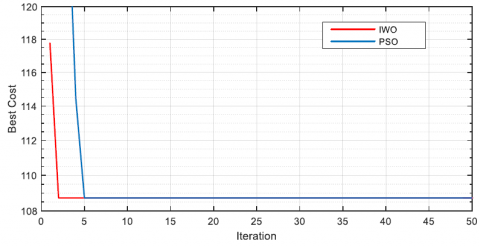Figure 13. Iterative convergence curve

## 1.14.pngFigure 14. The Type-2 membership function of fuzzy sets of inputs interval Type-2 fuzzy logic controller after tuning by the IWO (the FOU size parameter U=0.8534)

Simulation results show:

The Invasive weed optimization (IWO) algorithm converges quicker than the Particle Swarm Optimization algorithm (PSO) (Figure 13 and Figure 16). The superiority of the IT2F-PID controller with IWO comparing with both the IT2F-PID controller with PSO and fuzzy Logic PID controllers(Figure 15, Figure 18, Table 6 and Table 8), where: the IT2F-PID controller with IWO has minimum the Rise time (Tr), achieve lower the integral of square of errors (ISE) and the integral of the absolute errors (IAE) comparing with the other controllers. Finally; Invasive Weed Optimization Algorithm is helpful to tuning the PID values and the footprint of uncertainty size (FOU) in Interval Type-2 fuzzy logic PID controller for Boost DC-DC Converter.

## 1.15.pngFigure 15. The output voltage of different simulation cases

## 1.16.pngFigure 16. Iterative convergence curve

## 1.17.pngFigure 17. The Type-2 membership function of fuzzy sets of inputs Interval Type-2 fuzzy logic controller after tuning by the IWO (the FOU size parameter U=0.8219)

## 1.18.png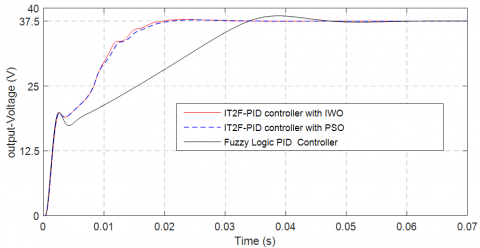Figure 18. The output voltage of different simulation cases

References

 Zadeh, L.A. (1975). The concept of a linguistic variable and its application to approximate reasoning-I. Information Sciences, 8(3): 199-249. https://doi.org/10.1016/0020-0255(75)90036-5

 Mendel, J. (2017). Uncertain Rule-Based Fuzzy Systems Introduction and New Directions, 2nd Edition. Springer International Publishing AG 2017. https://doi.org/10.1007/978-3-319-51370-6

 Wu, D., Mendel, J.M. (2007). Uncertainty measures for interval Type-2 fuzzy sets. Information Sciences, 177(23): 5378-5393. https://doi.org/10.1016/j.ins.2007.07.012

 Zhou, H., Ying, H., Zhang, C. (2019). Effects of increasing the footprints of uncertainty on analytical structure of the classes of interval Type-2 Mamdani and TS fuzzy controllers. IEEE Transactions on Fuzzy Systems, 27(9). https://doi.org/10.1109/TFUZZ.2019.2892354

 Sepulveda, R., Melin, P., Rodriguez, A., Mancilla, A., Montiel. O. (2006). Analyzing the effects of the footprint of uncertainty in Type-2 fuzzy logic controllers. Engineering Letters, 13(2).

 Aladi, J.H., Wagner, C., Garibaldi, M.J., Pourabdollah, A. (2015). On transitioning from Type-1 to interval Type-2 fuzzy logic systems. IEEE International Conference on Fuzzy Systems (FUZZ-IEEE). https://doi.org/10.1109/FUZZ-IEEE.2015.7338004

 Martinez, J.S., Péra, M.C., Hissel, D. (2012). Type-2 fuzzy logic control of a DC/DC buck converter. Elsevier, IFAC Proceedings Volumes, 45(21): 103-108. https://doi.org/10.3182/20120902-4-FR-2032.00020

 Bennaoui, A., Saadi, S. (2017). Type-2 fuzzy logic PID controller and different uncertainties design for boost DC-DC converters. Springer, Electrical Engineering, 99(1): 203-211. https://doi.org/10.1007/s00202-016-0412-3

 Vidal-Ldiarte, E., Martine-Salamero, L., Guinjoan, F., Calvente, J., Gomariz, S. (2004). Sliding and fuzzy control of a boot converter using an 8-bit microcontroller. IEE Proceedings - Electric Power Applications, 151(1): 5-11. https://doi.org/10.1049/ip-epa:20040086

  Middlebrook, R.D., Cuk, S. (2006). Advances in Switched-Mode Power Conversion. Pasadena (California): TESLAco, cop.

 Vorperian, V. (1990). Simplified analysis of PWM converters using model of PWM switch part I and part II. IEEE Transactions on Aerospace and Electronic Systems, 26(3): 490-496. https://doi.org/10.1109/7.106126

 Lin, P.Z., Hsu, C.F., Lee, T.T. (2005). Type-2 fuzzy logic controller design for buck DC-DC converters. IEEE Xplore, The 14th IEEE International Conference on Fuzzy Systems, Reno, NV, USA. https://doi.org/10.1109/FUZZY.2005.1452421

 Krein, P.T., Bentsman, J., Bass, R.M., Lesieutre, B.L. (1990). On the use of averaging for the analysis of power electronic systems. IEEE Transactions on Power Electronics, 5(2): 182-190. https://doi.org/10.1109/63.53155

 Lynch, C., Hagras, H., Callaghan, V. (2006). Using uncertainty bounds in the design of an embedded realtime Type-2 neuro-fuzzy speed controller for marine diesel engines. IEEE International Conference on Fuzzy Systems Sheraton Vancouver Wall Centre Hotel, Vancouver, BC, Canada, pp. 1446-1453. https://doi.org/10.1109/FUZZY.2006.1681899

 John, R., Hagras, H., Castillo, O. (2018). Type-2 fuzzy logic and systems. International Publishing AG. Studies in Fuzziness and Soft Computing, 362. https://doi.org/10.1007/978-3-319-72892-6

 Fan, Q.F., Wang, T., Chen, Y., Zhang, Z.F., (2018). Design and application of interval Type-2 tsk fuzzy logic system based on QPSO algorithm. International Journal of Fuzzy Systems, 20(3): 835–846. https://doi.org/10.1007/s40815-017-0357-3

 Boumella, N., Djouani, K., Boulemden, M. (2012). A robust interval Type-2 TSK Fuzzy Logic System design based on Chebyshev fitting. Int. J. Control Autom. Syst., 10(4): 727–736. https://doi.org/10.1007/s12555-012-0408-3

 Mehrabian, A.R., Lucas, C. (2006). A novel numerical optimization algorithm inspired from weed colonization. Ecological Informatics, 1(4): 355-366. https://doi.org/10.1016/j.ecoinf.2006.07.003

 Xing, B., Gao, W.J. (2014). Invasive Weed Optimization Algorithm. In: Innovative Computational Intelligence: A Rough Guide to 134 Clever Algorithms. Intelligent Systems Reference Library, vol 62. Springer, Cham.

 Nagaraju, T.V., Prasad, D.C., Murthy, N.G.K. (2019). Invasive Weed Optimization Algorithm for Prediction of Compression Index of Lime-Treated Expansive Clays. Springer, Singapore, Soft Computing for Problem Solving, pp. 317-324. https://doi.org/10.1007/978-981-15-0184-5_28

 Mandava, R.K., Vundavilli, P.R. (2018). Implementation of modified chaotic invasive weed optimization algorithm for optimizing the PID controller of the biped robot. Sādhanā, 43(66). https://doi.org/10.1007/s12046-018-0851-9

 Goli, A., Tirkolaee, E.B., Malmir, B., Bian, G.B., Sangaiah, A.K. (2019). A multi-objective invasive weed optimization algorithm for robust aggregate production planning under uncertain seasonal demand. Computing, 101: 499–529. https://doi.org/10.1007/s00607-018-00692-2

 Zhao, X.Q., Zhou, J.H. (2015). Improved kernel possibilistic fuzzy clustering algorithm based on invasive weed optimization. Journal of Shanghai Jiaotong University (Science), 20(2): 164-170. https://doi.org/10.1007/s12204-015-1605-z

 Mohanty, P.K., Parhi, D.R. (2014). A new efficient optimal path planner for mobile robot based on Invasive Weed Optimization algorithm. Front. Mech. Eng., 9: 317–330. https://doi.org/10.1007/s11465-014-0304-z

 Misaghi, M., Yaghoobi, M. (2019). Improved invasive weed optimization algorithm (IWO) based on chaos theory for optimal design of PID controller. Journal of Computational Design and Engineering, 6(3): 284-295. https://doi.org/10.1016/j.jcde.2019.01.001

 Guesmi, K., Essounbouli, N., Hamzaoui, A. (2008). Systematic design approach of fuzzy PID stabilizer for DC–DC converters. Elsevier, Energy Conversion and Management, 49(10): 2880-2889. https://doi.org/10.1016/j.enconman.2008.03.012

 Hissel, D. (1998). Contribution à la commande de dispositifs électrotechniques par logique floue Procédures de réglage sur site parplans d’expériences et méthodologie Taguchi, Doctoral Thesis, Institut National Polytechnique de Toulouse.